TECH INFOArduino入门指南

# 利用Arduino实现DIY伺服相机云台

### 目录

1. 水平相机支架简介
2. 设备规格
3. 3D 打印安装部件
4. 编写程序
5. 测试！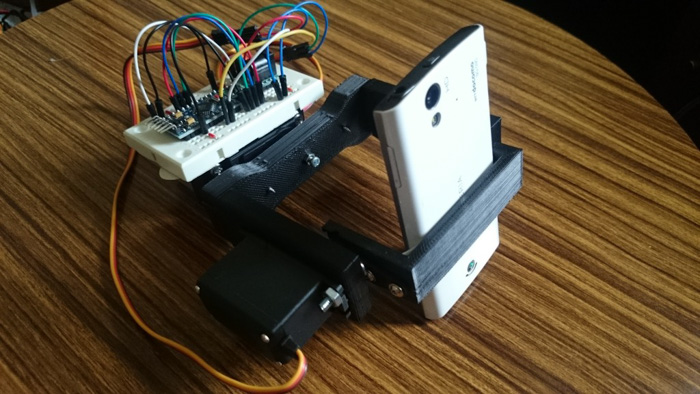## 1. 水平相机支架简介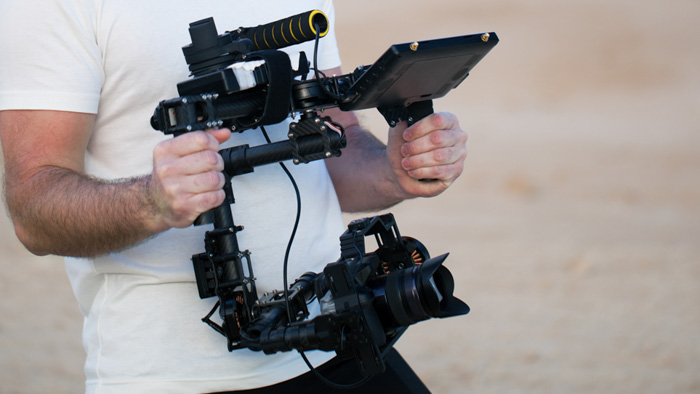## 2. 设备规格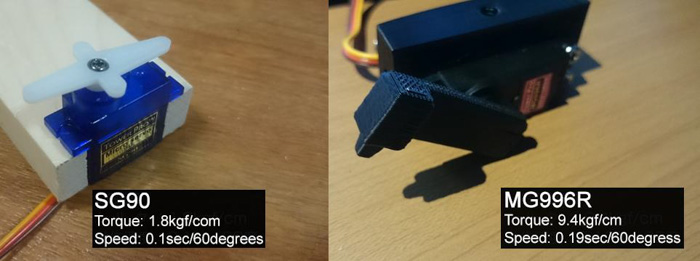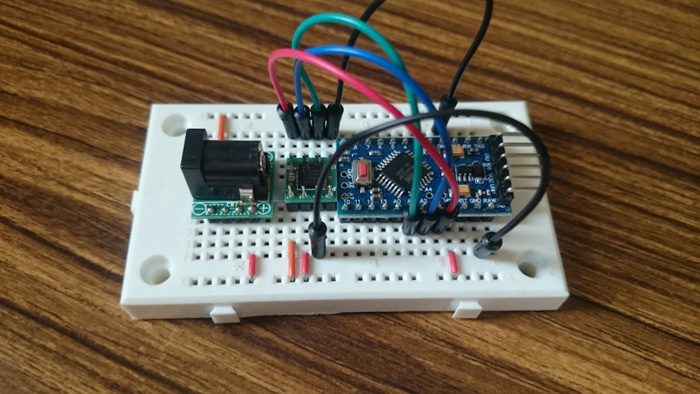## 3. 3D 打印安装部件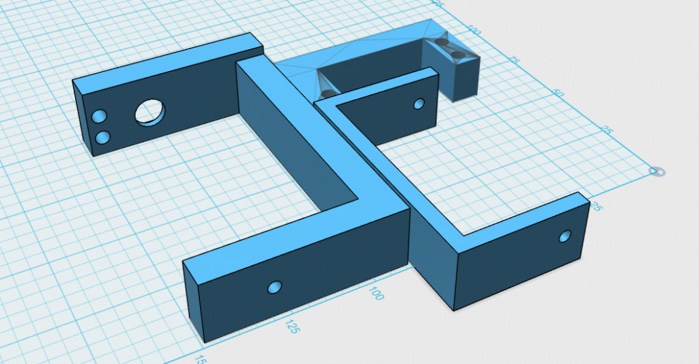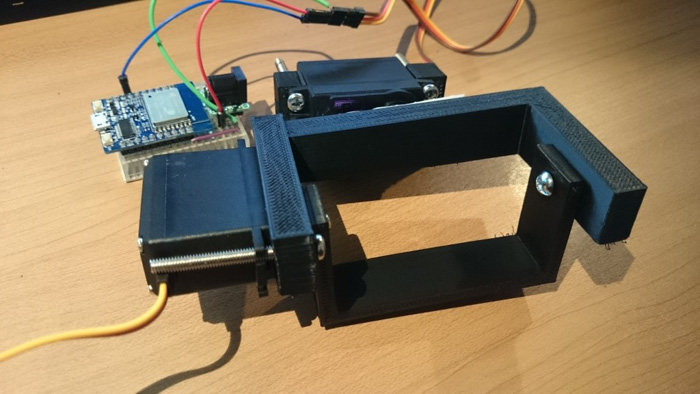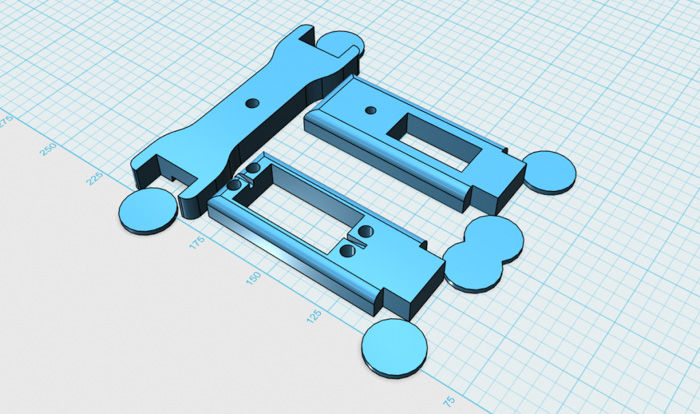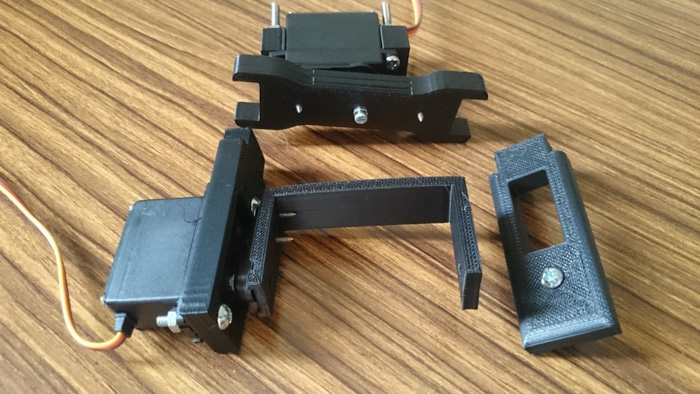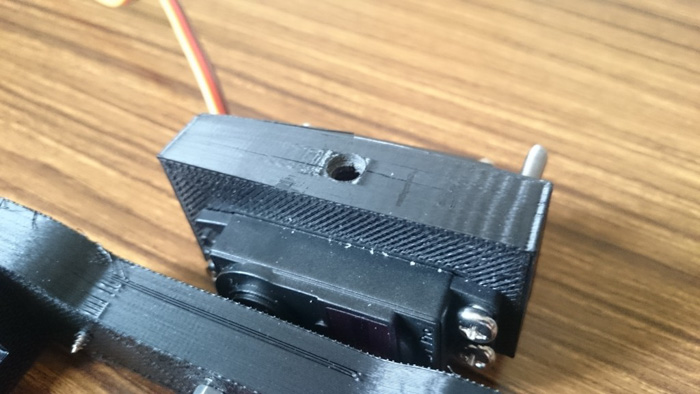## 4. 编写程序

``````int _maxX = 0;
int _minX = 1000;
int _maxY = 0;
int _minY = 1000;
int _maxZ = 0;
int _minZ = 1000;

//******************************************
//Program for acquiring accelerometer values //******************************************
void setup()
{
// initialize the serial monitor
Serial.begin(9600) ;
}
void loop()
{
long x , y , z ;
x = y = z = 0 ;
x = analogRead(1) ; // Ｘ axis
y = analogRead(2) ; // Ｙ axis
z = analogRead(3) ; // Ｚ axis

if(_maxX < x){ _maxX = x; }
if(_minX > x){ _minX = x; }
if(_maxY < y){ _maxY = y; }
if(_minY > y){ _minY = y; }
if(_maxZ < z){ _maxZ = z; }
if(_minZ > z){ _minZ = z; }

int centerX = (_maxX-_minX)/2+_minX;
int centerY = (_maxY-_minY)/2+_minY;
int centerZ = (_maxZ-_minZ)/2+_minZ;

float rotateX = ((float)_maxX-(float)_minX)/180;
float rotateY = ((float)_maxY-(float)_minY)/180;
float rotateZ = ((float)_maxZ-(float)_minZ)/180;

Serial.print("X:") ;
Serial.print(_minX) ;
Serial.print(" ") ;
Serial.print(centerX) ;
Serial.print(" ") ;
Serial.print(_maxX) ;
Serial.print(" ") ;
Serial.print(rotateX) ;
Serial.print(" Y:") ;
Serial.print(_minY) ;
Serial.print(" ") ;
Serial.print(centerY) ;
Serial.print(" ") ;
Serial.print(_maxY) ;
Serial.print(" ") ;
Serial.print(rotateY) ;
Serial.print(" Z:") ;
Serial.print(_minZ) ;
Serial.print(" ") ;
Serial.print(centerZ) ;
Serial.print(" ") ;
Serial.print(_maxZ) ;
Serial.print(" ") ;
Serial.println(rotateZ) ;
delay(50) ;
}
``````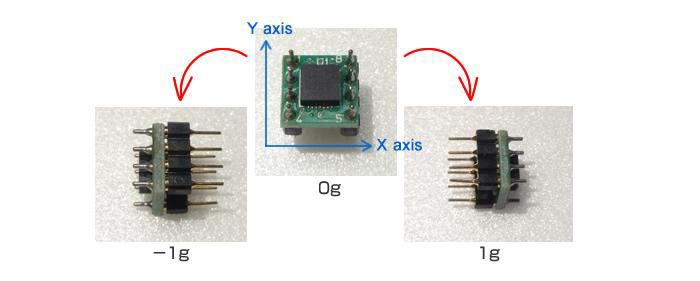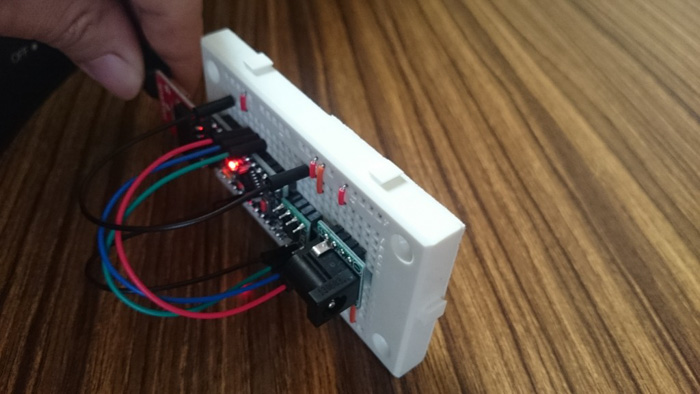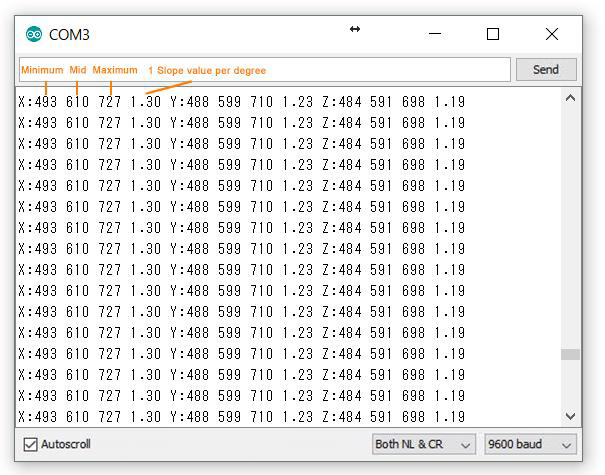``````//********************************************
//Program 2 for acquiring accelerometer values
//********************************************
#include <Servo.h>
Servo myservoX;
Servo myservoY;

void setup()
{
// initialize the serial monitor
Serial.begin(9600) ;
myservoX.attach( 5 );
myservoY.attach( 6 );
}

void loop()
{
int i ;
long x , y;
// read each data 100 times and average
x = y = 0 ;
for (i=0 ; i < 100 ; i++) {
}

x = x / 100 ;
y = y / 100 ;

int rotateX = (x-493)/1.30; //obtain anble
myservoX.write( 90-rotateX );
Serial.print("X:") ;
Serial.print(x) ;
Serial.print(" ") ;
Serial.print(rotateX) ;

int rotateY = (y-488)/1.23;
myservoY.write( 90-rotateY );
Serial.print(" Y:") ;
Serial.print(y) ; // Y axis
Serial.print(" ") ;
Serial.println(rotateY) ; // Y axis
delay(10) ;
}
``````

## 5. 测试！